# 1. 前言¶

PFLD全称A Practical Facial Landmark Detector是一个精度高，速度快，模型小的人脸关键点检测模型。在移动端达到了超实时的性能（模型大小2.1Mb，在Qualcomm ARM 845 处理器上达到140fps），作者分别来自武汉大学，天津大学，腾讯AI Lab，美国天普大学，有较大的实用意义。

# 2. 挑战¶

• 局部变化：现实场景中人脸的表情，广告，以及遮挡情况都有较大的变化，如Figure1所示
• 全局变化：姿态和成像质量是影响图像中人脸的表征的两个主要因素，人脸全局结构的错误估计将直接导致定位不准
• 数据不平衡：不平衡的数据使得算法模型无法正确表示数据的特征
• 模型的性能：由于手机和嵌入式设备计算性能和内存资源的限制，必须要求检测模型的size小处理速度快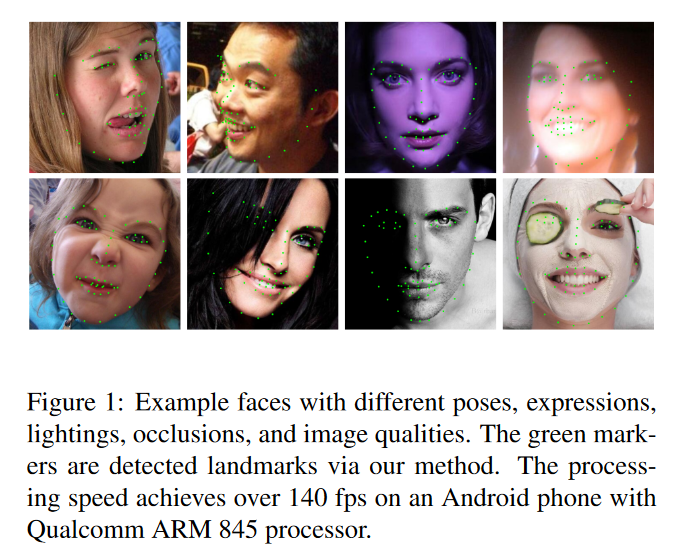# 4. PFLD网络结构¶

PFLD的网络结构如下图所示：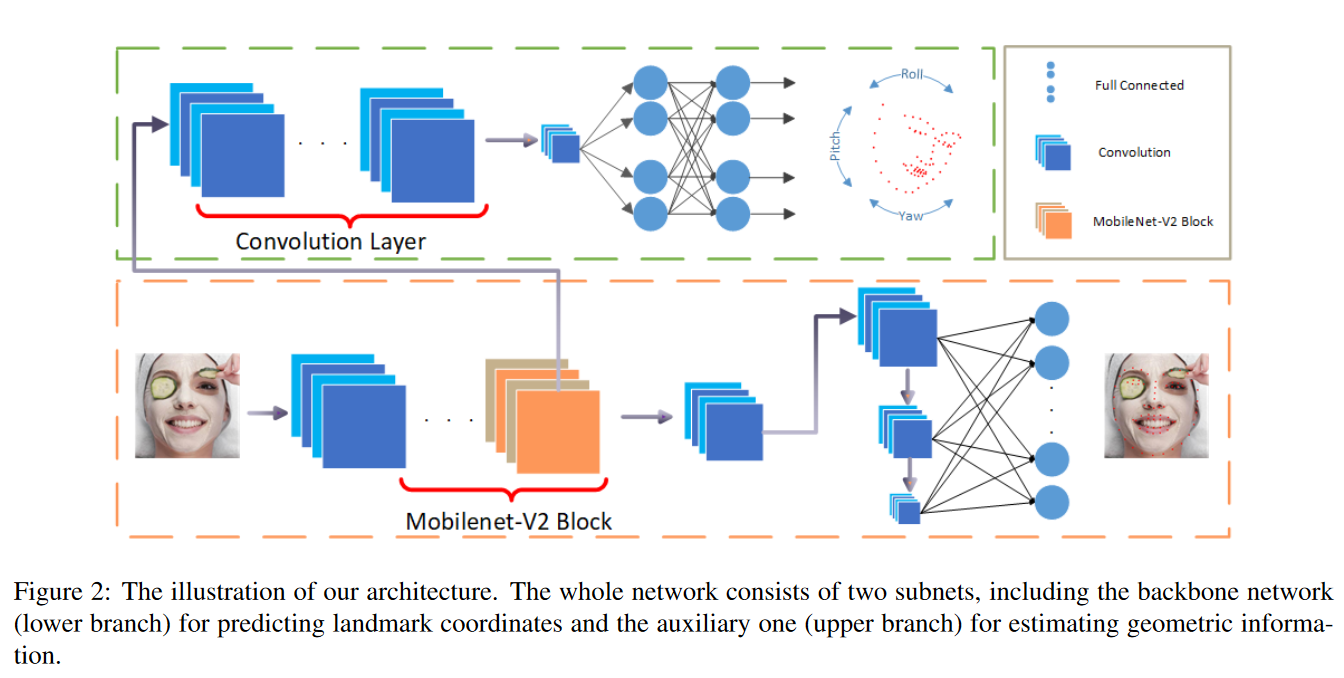## 4.1 损失函数设计¶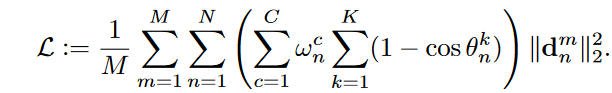• $\sum_{c=1}^Cw_n^c\sum_{k=1}^K(1-cos\theta_{n}^k)$代表权重$\gamma_n$
• $\theta^1$,$\theta^2$,$\theta^3$ ($K=3$)分别表示GT和Prediction在yaw、pitch、roll三种角度之间的偏差，角度越大$cos$值越小，权重越大。其中pitch代表上下翻转，yaw代表水平翻转，roll代表平面内旋转，都表示人脸的一种姿态。
• $C$表示不同的类别的人脸: 正脸、侧脸、抬头、低头、表情以及遮挡情况，$w_n^c$根据样本类别分数进行调整，论文中使用的分数样本数的导数计算的。
• $d_n^m$由主分支网络计算得到，$\theta_n^k$由辅助网络计算得到，然后由Loss来建立联系。

## 4.2 辅助网络的细节¶

PFLD在训练过程中引入了一个辅助网络用以监督PFLD网络模型的训练，如Figure2中绿色虚线里的部分。该子网络仅在训练的阶段起作用，在推理阶段不起作用。

## 4.3 主分支网络和辅助网络的详细配置¶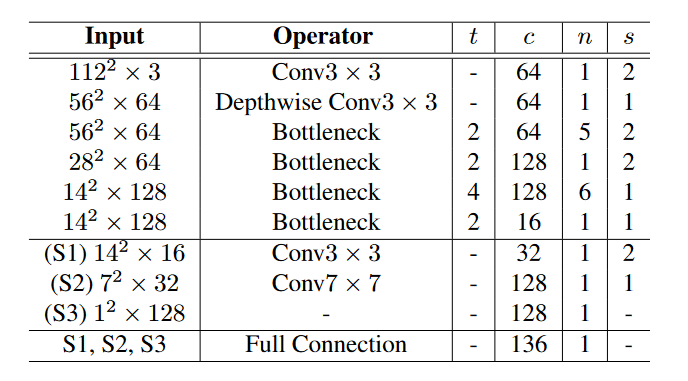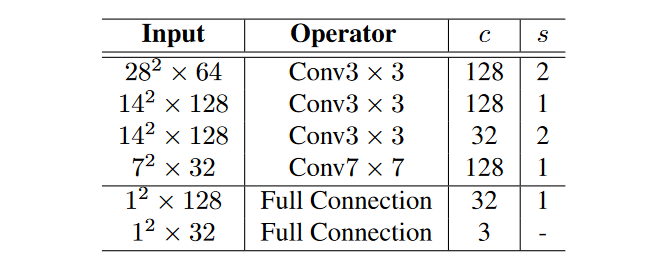# 5. 实验结果¶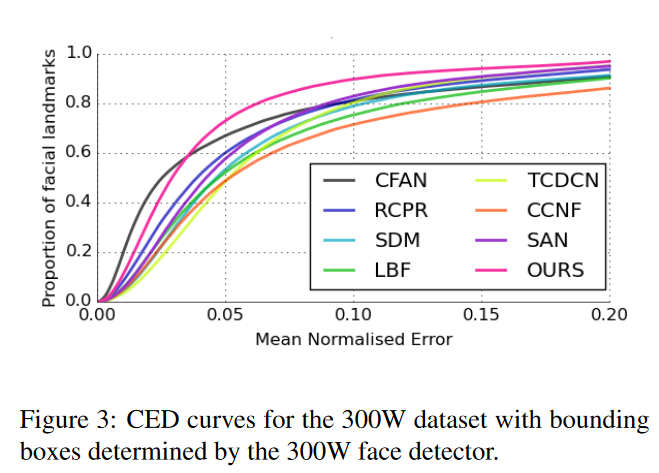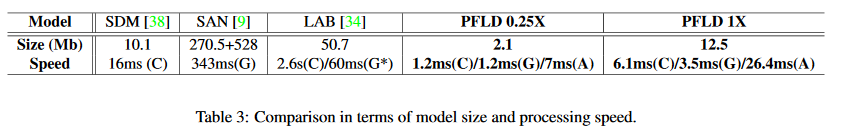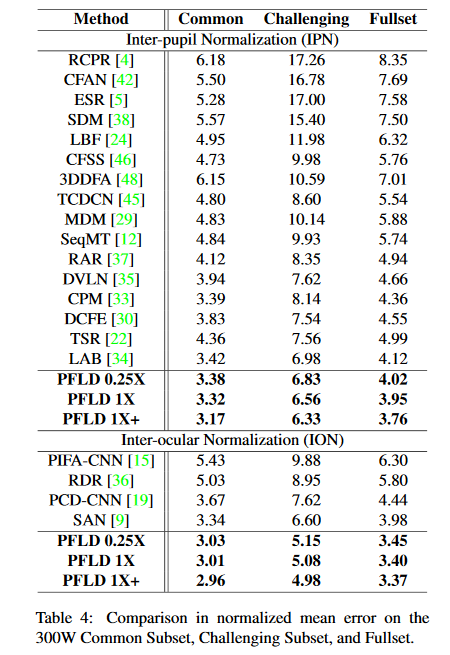Table5还展示了FPLD在AFLW数据集上的表现：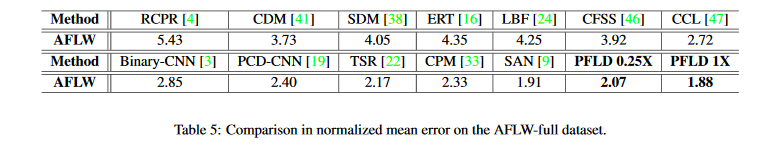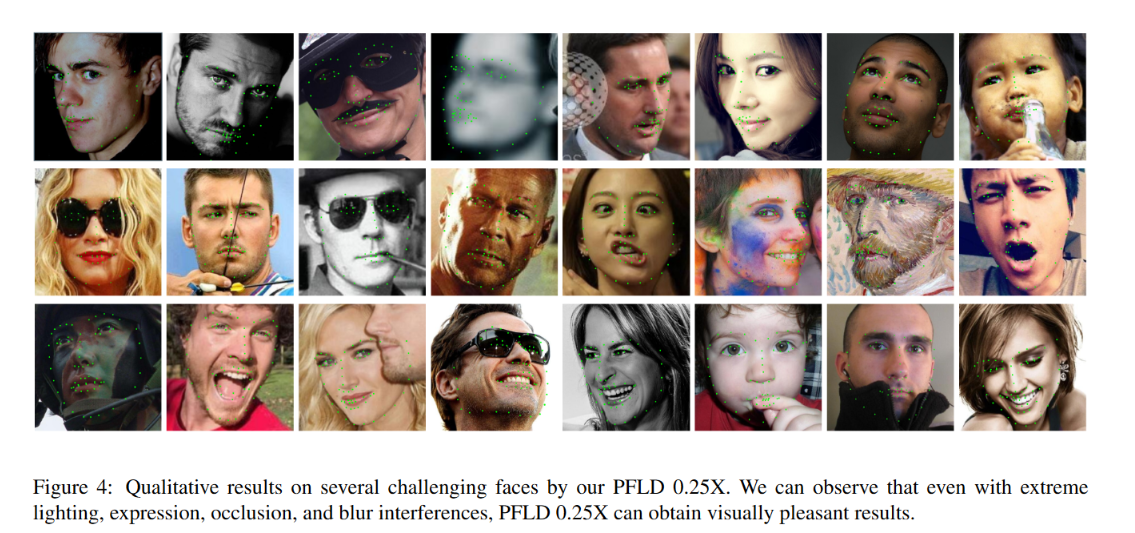# 7. 参考文章¶